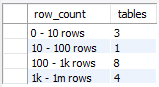## Number of tables by the number of rows in MySQL database

Article for:

If you want to get an overview of the number of rows the tables contain in your database (schema), one way to count them is by intervals of rows. This query returns the number of tables grouped by the number of rows at predefined intervals.

## Notes

Some storage engines, such as MyISAM, store the exact count. For other storage engines, such as InnoDB, this value is an approximation, and may vary from the actual value by as much as 40% to 50%.

## Query

``````select row_count,
count(table_name) as tables
from (
select concat(table_schema, '.', table_name) as table_name,
case when table_rows > 1000000000 then '1b rows and more'
when table_rows > 1000000 then '1m - 1b rows'
when table_rows > 1000 then '1k - 1m rows'
when table_rows > 100 then '100 - 1k rows'
when table_rows > 10 then '10 - 100 rows'
else  '0 - 10 rows' end as row_count
from information_schema.tables
where table_schema not in ('information_schema', 'sys',
'performance_schema', 'mysql')
and table_type = 'BASE TABLE'
-- and table_schema = 'database_name' -- put your database name here
) as tables_rows_interval
group by row_count
order by row_count;
``````

## Columns

• row_count - predefined row count intervals:
• 0 - 10 rows
• 10 - 100 rows
• 100 - 1k rows
• 1k - 1m rows
• 1m - 1b rows
• 1b rows and more
• tables - number of tables whose row count falls in that interval

## Rows

• One row: represents one interval
• Scope of rows: all row count intervals that appear in the database (schema)
• Ordered by: from the smallest table row count to the largest

## Sample results

Below is a number of tables grouped by row count in predefined intervals for the Sakila database (schema).